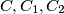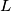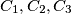### IMO Shortlist 1982 problem 12

Kvaliteta:
Avg: 0,0
Težina:
Avg: 0,0
Four distinct circles$C,C_1, C_2$, C3 and a line L are given in the plane such that$C$ and$L$ are disjoint and each of the circles$C_1, C_2, C_3$ touches the other two, as well as$C$ and$L$. Assuming the radius of$C$ to be$1$, determine the distance between its center and$L.$
Izvor: Međunarodna matematička olimpijada, shortlist 1982